## Nickzom Calculator Solves Calculations on Gas Laws

In Physics and Chemistry, there are four prominent gas laws that govern calculations related to gases. These laws have major parameters such as:

• Pressure
• Temperature
• Volume

The four gas laws are:

• Boyle’s Law
• Charles’ Law
• Pressure or Gay Lussac’s Law
• The General Gas Equation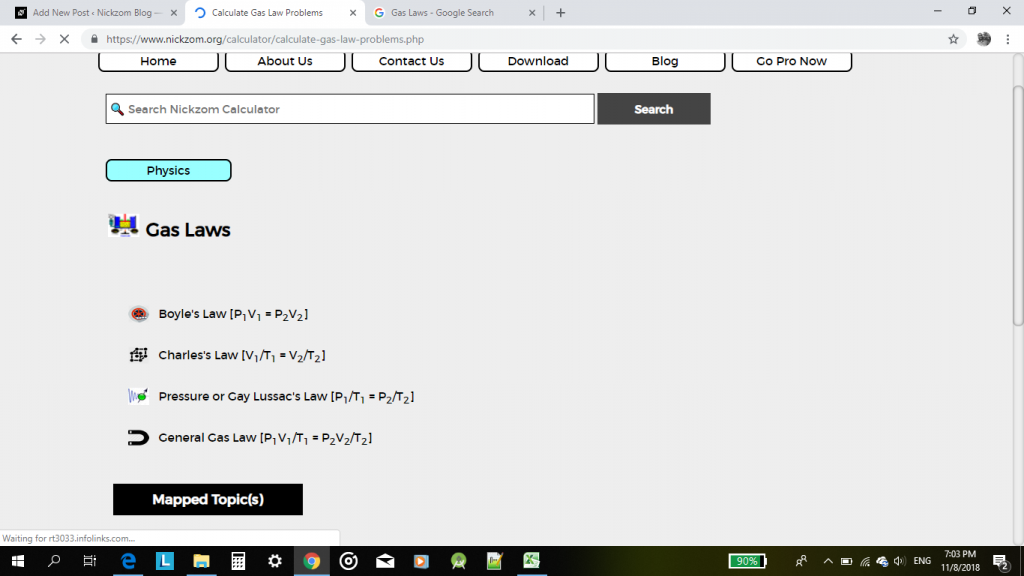According to Boyle’s law, pressure is indirectly proportional to volume.

According to Charles’ law, volume is directly proportional to temperature.

According to Pressure or Gay Lussac’s law, pressure is directly proportional to temperature.

According to the General Gas Equation or law, the product of pressure and volume is directly proportional to temperature.

For this post, I would be given an example of how Nickzom Calculator can solve a problem on gas laws with the general gas equation or law.

One can access Nickzom Calculator via any of these channels:

Let’s assume that one is looking for an unknown temperature.

First and foremost, click on General Gas Law.

Then click on Unknwon Temperature.Now, proceed to enter the values of the known parameters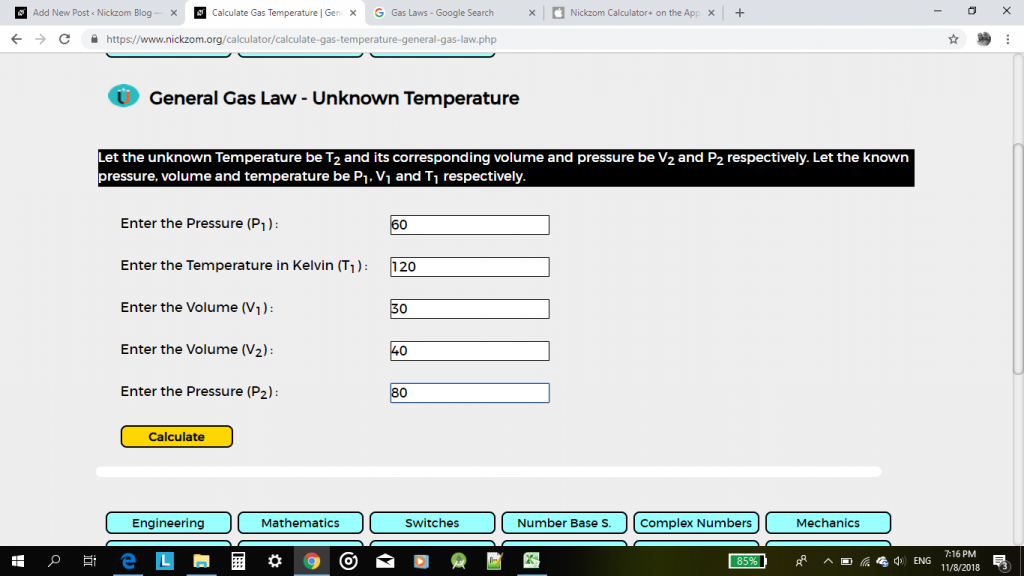Then, click on calculate.

The result would be displayed for you in less than a second.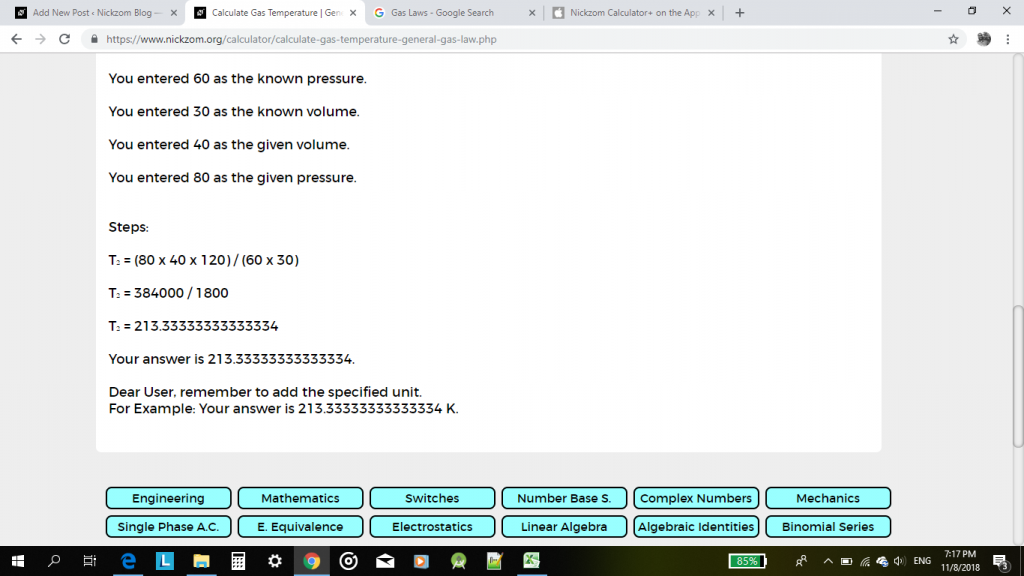This pattern can be used to solve any calculation problem one faces in gas laws.

## Physics, Elasticity Calculations Solutions By Nickzom Calculator – The Calculator Encyclopedia

According to Wikipedia,

In Physics, elasticity is the ability of a body to resist a distorting influence and to return to its original size and shape when that influence or force is removed. Solid objects will deform when adequate forces are applied to them. If the material is elastic, the object will return to its initial shape and size when these forces are removed.

At Nickzom Calculator, we solve for the following:

1. Hooke’s Law Parameters (Force, Extension and Elastic Constant)
2. Young’s Modulus
3. Stress
4. Strain
5. Work Done or Elastic Potential Energy (Force)
6. Work Done or Elastic Potential Energy (Elastic Constant)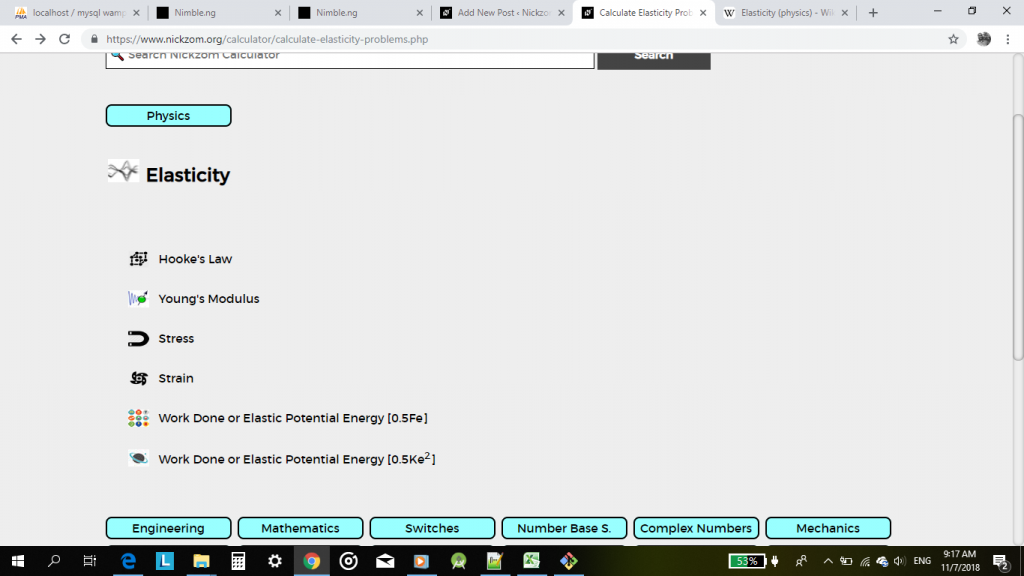Let’s say for example you want to find the force of an elastic material using Hooke’s law.

First, you need to get Nickzom Calculator either on web, or as an app via this links

Apple (iTunes – Paid) – https://itunes.apple.com/us/app/nickzom-calculator/id1331162702?mt=8

Next, you go to Physics, Then click on Elasticity.

Once you click on Elasticity, a new page comes up, click on Hooke’s lawEnter the elastic constant (K) and the extension for the elastic material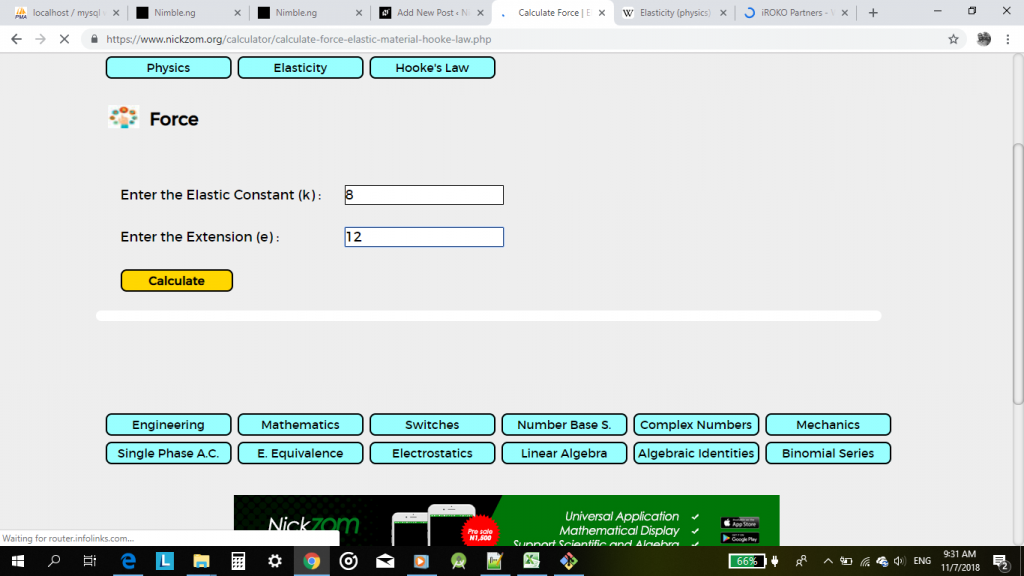Once, you have entered the values click on calculate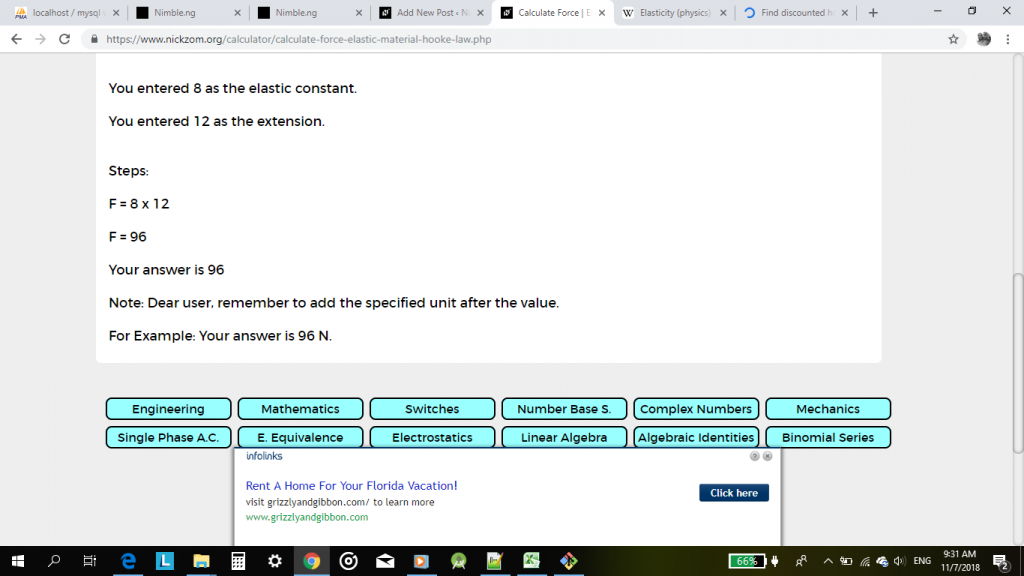As you can see above. Nickzom Calculator would solve the problems and provide you with a valid answer alongside the workings.

You can also apply the same steps in calculating for problems in Young’s modulus, stress, strain and work done.

## The Calculator For Physicists

Nickzom Calculator+ is a 100% all in one package that solves over 32,000 calculations and shows the steps of the calculations. One of the sections it handles is Physics.
Nickzom Calculator+ solves over 8,500+ calculations in Physics and shows the steps, formulas, parameters and answer accurately and comprehensively.

Nickzom Calculator+ handles the following major chapters of Physics:

– Elasticity
– Electric Field
– Electrolysis
– Electromagnetic Field
– Energy Quantization
– Equilibrium of Forces
– Gas Laws
– Gravitational Field
– Heat Energy
– Machines
– Magnetic Field
– Motion
– Pressure
– Projectile
– Resistivity
– Simple A. C. Circuit
– Vector Resultant
– Waves
– Wave – Particle Behaviour
– Work, Energy and Power

Within these major topics there are sub topics right up to selections of the various parameters you desire to find.

To obtain this calculator via web

Visit https://www.nickzom.org/calculator
Scroll to the Bottom
Click on Access Our Professional Version
Register with Us
Make a Payment of NGN 1,500
Select Physics in the Drop Down
Click on “Open Section”

To obtain this calculator via Google Play Store (For Android)